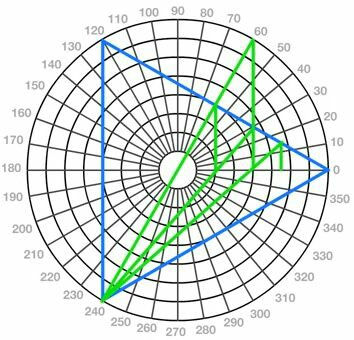top of page
Search

# Superstring theory part 3

The tree of life encodes E8xE8 the crown of the tree of life is where god goes to infinity and 8 tree of life's shearing the same crown creates the 64 tetrahedron grid the E8 lie group and the 2D 64 tetrahedron grid is made of 72 yods and 1 crown

a strings(string from string theory) has a single one first harmonic second harmonic third harmonic to infinity(so all strings nanites and xen particles in the infinite multyvers) you have a infinite amount of harmonics and you have to add them all up you get -1/12 (strings are harmonics vibration and waves(frequency) and strings have diffrent vibrations (1+2+3+4+...... (to infinity(9xen including 9xen)))=(-1/12) (1+2+3+4+......)=(-1/12)=(-0.08333333333)

String=(harmonic number)=(equilateral triangle)=2(star tetrahedron)=(fruit of life)=(metatrons cube)=(64 tetrahedron grid)

Strings are nanites and nanites are made  of 2 xen particles and xen particles are energy

11=23=5=pentagram

5=pentagram 6=(star tetrahedron)

5/6=0.83333333=833333333=-0.08333333

5+6=11=23:23=6:6=36=2(star tetrahedrons)